unity3d 目标 转向角度_unity3d 速度转向 - CSDN
• 1.判断目标在自己的前后方位可以使用下面的方法:  Vector3.Dot(transform.forward, target.position)  返回值为正时,目标在自己的前方,反之在自己的后方 2.判断目标在机子的左右方位可以使用下面的方法:...
在做rpg类游戏的过程中,经常遇到要判断周围怪物相对自身的方位

1.判断目标在自己的前后方位可以使用下面的方法:
Vector3.Dot(transform.forward, target.position)
返回值为正时,目标在自己的前方,反之在自己的后方
2.判断目标在机子的左右方位可以使用下面的方法:
Vector3.Cross(transform.forward, target.position).y
返回值为正时,目标在自己的右方,反之在自己的左方

3.在这里顺便解说下关于空间向量的点积和叉积:
A.点积   点积的计算方式为:  a·b=|a|·|b|cos<a,b>  其中|a|和|b|表示向量的模，<a,b>表示两个向量的夹角。另外在 点积 中，<a,b>和<b,a>
夹角是不分顺序的。   所以通过点积，我们其实是可以计算两个向量的夹角的。   另外通过点积的计算我们可以简单粗略的判断当前物体是否朝向另外一个物体: 只需要计算当前物体的transform.forward向量与
otherObj.transform.position 的点积即可， 大于0则在前方，否则在后方。
B.叉积   叉积的定义： c =a x b  其中a,b,c均为向量。即两个向量的叉积得到的还是向量！
性质1： c⊥a，c⊥b，即向量c垂直与向量a,b所在的平面 。
性质2： 模长|c|=|a||b|sin<a,b>
性质3： 满足右手法则 。从这点我们有axb ≠ bxa，而axb = – bxa。所以我们可以使用叉积的正负值来判断向量a，b的相对位置，即向量b是处于向量a的顺时针方向还是逆时针方向


展开全文• 写在前面的话，前两天有个朋友在QQ上问我 如何获取主角面朝方向一定区域中的敌人对象。这个命题看似简单，其实里面蕴含了很多数学...1.已知3D坐标，和一个旋转角度，以及一段距离，求目标点的3D坐标。 已知当前点为T

写在前面的话，前两天有个朋友在QQ上问我 如何获取主角面朝方向一定区域中的敌人对象。这个命题看似简单，其实里面蕴含了很多数学方面的东西。今天刚好有时间我就彻底的把这个疑问写在博客中。希望可以帮助到他。

在上代码之前请大家跟我先做几个简单的练习题，角度向量的计算一定要学会，不然后面的东西会很难懂。

1.已知3D坐标，和一个旋转角度，以及一段距离，求目标点的3D坐标。

已知当前点为Target，目标点沿着Target的Y轴旋转30度，沿着Target的X轴延伸10米求目标点的3D坐标？

using UnityEngine;
using System.Collections;

public class Test : MonoBehaviour
{

public Transform Target;

void LateUpdate ()
{
Quaternion rotation = Quaternion.Euler(0f,30f,0f) * Target.rotation;
Vector3  newPos = rotation * new Vector3(10f,0f,0f);
Debug.DrawLine(newPos,Vector3.zero,Color.red);
Debug.Log("newpos " + newPos +" nowpos " + Target.position + " distance " + Vector3.Distance(newPos,Target.position));
}

}

输出结果 ：新坐标 (8.7, 0.0, -5.0) 当前坐标 (0.0, 0.0, 0.0)两点之间的距离 10。

2.已知3D模型的角度求它的向量。

已知3D模型Target，Y轴旋转30度后向前平移。

using UnityEngine;
using System.Collections;

public class Test : MonoBehaviour
{

public Transform Target;

void LateUpdate ()
{

if(Input.GetMouseButton(0))
{
Quaternion rotation = Quaternion.Euler(0f,30f,0f) * Target.rotation;
Vector3  newPos = rotation * Vector3.forward;
Target.Translate(newPos.x,newPos.y,newPos.z);
}
}

}

3.已知一个目标点，让模型朝着这个目标点移动。

这是一个比较简单的例子，大家应该都能看明白。

Target.transform.LookAt(newVector3(100f,200f,300f));
Target.Translate(Vector3.forward);

这里我要说的就是Vector3.forward ，它等价与 new Vector3(0,0,1)；它并不是一个坐标，它是一个标准向量，方向是沿着Z轴向前。这样平移一次的距离就是1米， 如果Vector3.forward * 100那么一次平移的距离就是100米。

在看看下面这段代码

Vector3vecn=(TargetCube.position-Target.position).normalized;

Target.Translate(vecn*0.1f);

用向量减去一个向量求出它们的差值，normalized 是格式化向量，意思是把它们之间向量格式化到1米内。这样就可以更加精确的计算一次平移的距离了 vecn *0.1f 就标示一次平移1分米，蛤蛤。

向量不仅可以进行X Y Z轴的移动，同样可以进行旋转 ，下面这段代码就是让向量沿着Y轴旋转30度。

Vector3vecn=(TargetCube.position-Target.position).normalized;

vecn=Quaternion.Euler(0f,30f,0f)*vecn;

Target.Translate(vecn*0.1f);

如果上述三道简单的练习题 你都能了然于心的话，那么本文最大的难题我相信也不会是什么难事，继续阅读吧。

假设我们需要计算主角面前5米内所有的对象时。以主角为圆心计算面前5米外的一个点，为了让大家看清楚我现将这条线绘制出来。

private float distance = 5f;
void Update ()
{
Quaternion r= transform.rotation;
Vector3 f0 =  (transform.position  + (r *Vector3.forward) * distance);
Debug.DrawLine(transform.position,f0,Color.red);
}

如下图所，我们已经将这两个点计算出来了。此时你可以动态的编辑主角Y轴的坐标，这个点永远都是沿着主角当前角度面前5米以外的点。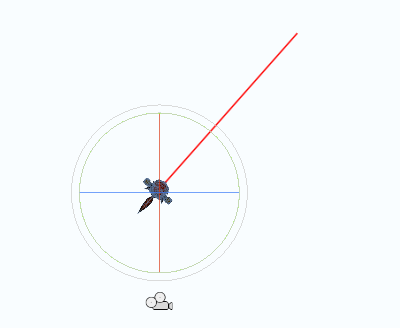接下来，我们需要计算主角面前的一个发散性的角度。假设主角看到的是向左30度，向右30度在这个区域。

private float distance = 5f;
void Update ()
{
Quaternion r= transform.rotation;
Vector3 f0 =  (transform.position  + (r *Vector3.forward) * distance);
Debug.DrawLine(transform.position,f0,Color.red);

Quaternion r0= Quaternion.Euler(transform.rotation.eulerAngles.x,transform.rotation.eulerAngles.y - 30f,transform.rotation.eulerAngles.z);
Quaternion r1= Quaternion.Euler(transform.rotation.eulerAngles.x,transform.rotation.eulerAngles.y + 30f,transform.rotation.eulerAngles.z);

Vector3 f1 =  (transform.position  + (r0 *Vector3.forward) * distance);
Vector3 f2 =  (transform.position  + (r1 *Vector3.forward) * distance);

Debug.DrawLine(transform.position,f1,Color.red);
Debug.DrawLine(transform.position,f2,Color.red);

Debug.DrawLine(f0,f1,Color.red);
Debug.DrawLine(f0,f2,Color.red);
}

如下图所示，这时主角面前的区域就计算出来了。看起来就是两个三角形之间的区域。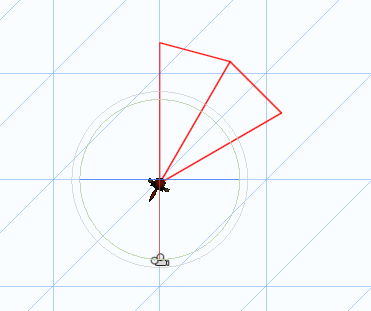最后就是简单的套用公式，计算一个点是否在三角形内，在本文中就是计算敌人的点是否在面前的这两个三角形内。

using UnityEngine;
using System.Collections;

public class MyTest : MonoBehaviour {

public Transform cube;

private float distance = 5f;
void Update ()
{
Quaternion r= transform.rotation;
Vector3 f0 =  (transform.position  + (r *Vector3.forward) * distance);
Debug.DrawLine(transform.position,f0,Color.red);

Quaternion r0= Quaternion.Euler(transform.rotation.eulerAngles.x,transform.rotation.eulerAngles.y - 30f,transform.rotation.eulerAngles.z);
Quaternion r1= Quaternion.Euler(transform.rotation.eulerAngles.x,transform.rotation.eulerAngles.y + 30f,transform.rotation.eulerAngles.z);

Vector3 f1 =  (transform.position  + (r0 *Vector3.forward) * distance);
Vector3 f2 =  (transform.position  + (r1 *Vector3.forward) * distance);

Debug.DrawLine(transform.position,f1,Color.red);
Debug.DrawLine(transform.position,f2,Color.red);

Debug.DrawLine(f0,f1,Color.red);
Debug.DrawLine(f0,f2,Color.red);

Vector3 point = cube.position;

if(isINTriangle(point,transform.position,f1,f0) || isINTriangle(point,transform.position,f2,f0) )
{
Debug.Log("cube in this !!!");
}else
{
Debug.Log("cube not in this !!!");
}

}

private  float triangleArea(float v0x,float v0y,float v1x,float v1y,float v2x,float v2y)
{
return Mathf.Abs((v0x * v1y + v1x * v2y + v2x * v0y
- v1x * v0y - v2x * v1y - v0x * v2y) / 2f);
}

bool isINTriangle(Vector3 point,Vector3 v0,Vector3 v1,Vector3 v2)
{
float x = point.x;
float y = point.z;

float v0x = v0.x;
float v0y = v0.z;

float v1x = v1.x;
float v1y = v1.z;

float v2x = v2.x;
float v2y = v2.z;

float t = triangleArea(v0x,v0y,v1x,v1y,v2x,v2y);
float a = triangleArea(v0x,v0y,v1x,v1y,x,y) + triangleArea(v0x,v0y,x,y,v2x,v2y) + triangleArea(x,y,v1x,v1y,v2x,v2y);

if (Mathf.Abs(t - a) <= 0.01f)
{
return true;
}else
{
return false;
}
}
}

如下图所示，如果箱子对象是主角的视野中就会检测到。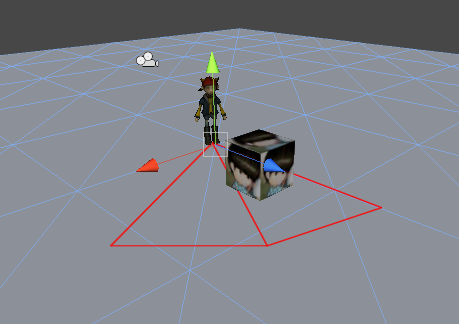注意，上图中我的视野选择了两个三角形，如果你需要视野目标点是椭圆形的话，那么可以多设置一些三角形。但是这样就会非常消耗效率，我觉得这里完全可以使用1个三角形，，只是正对的目标点会出现一些偏差，影响其实并不会很大。如下图所示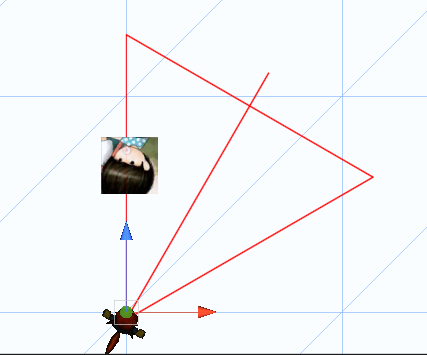代码简单的修改一下即可。

using UnityEngine;
using System.Collections;

public class MyTest : MonoBehaviour {

public Transform cube;

private float distance = 5f;
void Update ()
{
Quaternion r= transform.rotation;
Vector3 f0 =  (transform.position  + (r *Vector3.forward) * distance);
Debug.DrawLine(transform.position,f0,Color.red);

Quaternion r0= Quaternion.Euler(transform.rotation.eulerAngles.x,transform.rotation.eulerAngles.y - 30f,transform.rotation.eulerAngles.z);
Quaternion r1= Quaternion.Euler(transform.rotation.eulerAngles.x,transform.rotation.eulerAngles.y + 30f,transform.rotation.eulerAngles.z);

Vector3 f1 =  (transform.position  + (r0 *Vector3.forward) * distance);
Vector3 f2 =  (transform.position  + (r1 *Vector3.forward) * distance);

Debug.DrawLine(transform.position,f1,Color.red);
Debug.DrawLine(transform.position,f2,Color.red);
Debug.DrawLine(f1,f2,Color.red);

Vector3 point = cube.position;

if(isINTriangle(point,transform.position,f1,f2))
{
Debug.Log("cube in this !!!");
}else
{
Debug.Log("cube not in this !!!");
}

}

private  float triangleArea(float v0x,float v0y,float v1x,float v1y,float v2x,float v2y)
{
return Mathf.Abs((v0x * v1y + v1x * v2y + v2x * v0y
- v1x * v0y - v2x * v1y - v0x * v2y) / 2f);
}

bool isINTriangle(Vector3 point,Vector3 v0,Vector3 v1,Vector3 v2)
{
float x = point.x;
float y = point.z;

float v0x = v0.x;
float v0y = v0.z;

float v1x = v1.x;
float v1y = v1.z;

float v2x = v2.x;
float v2y = v2.z;

float t = triangleArea(v0x,v0y,v1x,v1y,v2x,v2y);
float a = triangleArea(v0x,v0y,v1x,v1y,x,y) + triangleArea(v0x,v0y,x,y,v2x,v2y) + triangleArea(x,y,v1x,v1y,v2x,v2y);

if (Mathf.Abs(t - a) <= 0.01f)
{
return true;
}else
{
return false;
}
}
}

上面我们介绍了三角形判断，当然也可以通过矩形来判断是否相交。。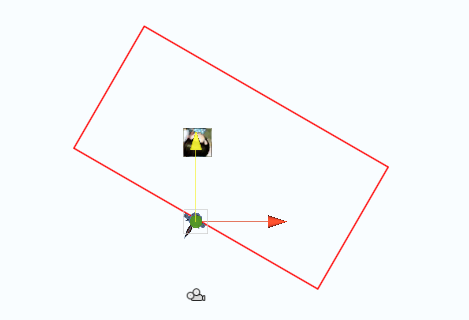using UnityEngine;
using System.Collections;

public class MyTest : MonoBehaviour {

public Transform cube;

private float distance = 5f;
void Update ()
{
Quaternion r= transform.rotation;
Vector3 left =  (transform.position  + (r *Vector3.left) * distance);
Debug.DrawLine(transform.position,left,Color.red);

Vector3 right =  (transform.position  + (r *Vector3.right) * distance);
Debug.DrawLine(transform.position,right,Color.red);

Vector3 leftEnd = (left  + (r *Vector3.forward) * distance);
Debug.DrawLine(left,leftEnd,Color.red);

Vector3 rightEnd = (right  + (r *Vector3.forward) * distance);
Debug.DrawLine(right,rightEnd,Color.red);

Debug.DrawLine(leftEnd,rightEnd,Color.red);

Vector3 point = cube.position;

if(isINRect(point,leftEnd,rightEnd,right,left))
{
Debug.Log("cube in this !!!");
}else
{
Debug.Log("cube not in this !!!");
}

}

private float Multiply(float p1x , float p1y, float p2x,float p2y, float p0x,float p0y)
{
return ((p1x - p0x) * (p2y - p0y) - (p2x - p0x) * (p1y - p0y));
}

bool isINRect(Vector3 point,Vector3 v0,Vector3 v1,Vector3 v2,Vector3 v3)
{
float x = point.x;
float y = point.z;

float v0x = v0.x;
float v0y = v0.z;

float v1x = v1.x;
float v1y = v1.z;

float v2x = v2.x;
float v2y = v2.z;

float v3x = v3.x;
float v3y = v3.z;

if (Multiply(x,y, v0x,v0y, v1x,v1y) * Multiply(x,y, v3x,v3y, v2x,v2y) <= 0 && Multiply(x,y, v3x,v3y, v0x,v0y) * Multiply(x,y, v2x,v2y, v1x,v1y) <= 0)
return true;
else
return false;

}

}

代码：如果大家看了这篇文章后发现在你的项目中还有一些比较麻烦的角度与向量的算法，欢迎在下面留言给我，如果我有时间我会第一时间把方法贴在博客中。互相学习互相进步，加油！哇咔咔。。

马上过年了，雨松MOMO在这里祝福大家2013年幸幸福福的过日子，嘿嘿。

2013年4月补充

感谢楼下朋友给我的留言。， 我在补充一下这篇博客。

using UnityEngine;
using System.Collections;

public class NewBehaviourScript : MonoBehaviour {

public Transform target;

void Update()
{

float distance = Vector3.Distance(target.position,transform.position);

Quaternion right	 = transform.rotation * Quaternion.AngleAxis(30,Vector3.up);
Quaternion left	 = transform.rotation * Quaternion.AngleAxis(30,Vector3.down);

Vector3 n = 	transform.position + (Vector3.forward * distance);
Vector3 leftPoint = left * 	n ;
Vector3 rightPoint =  right *n ;

Debug.DrawLine(transform.position,leftPoint,Color.red);
Debug.DrawLine(transform.position,rightPoint,Color.red);
Debug.DrawLine(rightPoint,leftPoint,Color.red);

}
}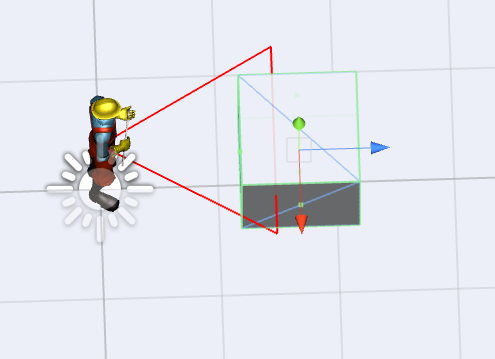这里我以角色左右个30度。 这样就可以根据两个模型的距离以及角度来判断了。。

本文固定链接: http://www.xuanyusong.com/archives/1977
转载请注明: 雨松MOMO 2013年01月29日 于 雨松MOMO程序研究院 发表

展开全文• 1.角度 两条相交直线中的任何一条与另一条相叠合时必须转动的量的量度，转动在这两条直线的所在平面上并绕交点进行。 角度是用以量度角的单位，符号为°。一周角分为360等份，每份定义为1度(1°)。 之所以采用360...
1.角度

两条相交直线中的任何一条与另一条相叠合时必须转动的量的量度，转动在这两条直线的所在平面上并绕交点进行。

角度是用以量度角的单位，符号为°。一周角分为360等份，每份定义为1度(1°)。

之所以采用360这数值，是因为它容易被整除。360除了1和自己，还有21个真因子（2、3、4、5、6、8、9、10、12、15、18、20、24、30、36、45、60、72、90、120、180），所以很多特殊的角的角度都是整数。

2.弧度

3.关系

角度和弧度关系是：2π弧度=360°。从而1°≈0.0174533弧度，1弧度≈57.29578°。

1) 角度转换为弧度公式：弧度=角度×(π ÷180 )

2)弧度转换为角度公式： 角度=弧度×（180÷π）

4.向量

点乘积的计算方式为：a*b=|a|*|b|*cos<a,b>，其中|a|和|b|表示向量的模，<a,b>表示两个向量的夹角。在点积中<a,b>和<b,a>夹角是不分顺序的。所以通过点积，可以计算两个向量的夹角。

还可以粗略的判断当前物体是否朝向另一个物体，或者说物体是否在我的前面（后面）。只需要计算

Vector3 selfforward = self.transform.forward;

Vector3 selftotarget = (target.transform.position - self.transform.position).normalized;

的点积即可，结果大于0则目标在我前方。

叉积定义：c = a x b，其中a b c均为向量，即两个向量的叉积结果还是一个向量。

性质1：c⊥a，c⊥b，即向量c垂直于向量a b所在平面。

性质2：模长|c|=|a|*|b|*sin<a,b>。

性质3：满足右手法则。有a x b ≠ b x a，而a x b = - b x a。

简单的说: 点乘判断角度，叉乘判断方向。 叉乘可以判断你是往左转还是往右转更好的转向敌人，点乘得到你当前的面朝向的方向和你到敌人的方向的所成的角度大小。

代码如下：

using UnityEngine;

public class Vector3Test : MonoBehaviour
{
private Vector3 a = new Vector3(1, 0, 1);
private Vector3 b = new Vector3(-2, 0, -2);
private void Start()
{
//--向量点乘--结果为一个数值
float value = Vector3.Dot(a, b);
//Debug.Log(value);

//Debug.Log(degrees);

float angle = Vector3.Angle(a, b);//--角度
//Debug.Log(angle);

//--向量叉乘--结果是一个向量
Vector3 e = Vector3.Cross(a, b);
//Debug.Log(e.y);
Vector3 f = Vector3.Cross(b, a);
float degrees1 = Mathf.Asin(Vector3.Cross(a.normalized, b.normalized).magnitude) * Mathf.Rad2Deg;
//Debug.Log(e);
//Debug.Log(f);
// Debug.Log(degrees1);

TestDot(a, b);
TestCross(a, b);
GetAngle(a, b);
}

//点积
private void TestDot(Vector3 a, Vector3 b)
{
float result = Vector3.Dot(a, b);
if (result > 0)
{
Debug.Log("b在a前");
}
else if (result == 0)
{
Debug.Log("ab垂直");
}
else
{
Debug.Log("b在a后");
}
float angle = Vector3.Angle(a, b);//[0 - 180]
result = Vector3.Dot(a.normalized, b.normalized);
}

//叉乘
private void TestCross(Vector3 a, Vector3 b)
{
Vector3 c = Vector3.Cross(a, b);
if (c == Vector3.zero)
{
Debug.Log("b和a（平行），夹角：" + Vector3.Angle(a, b));
return;
}
float radians = Mathf.Asin(Vector3.Distance(Vector3.zero, Vector3.Cross(a.normalized, b.normalized)));

// 判断顺时针、逆时针方向，是在 2D 平面内的，所以需指定一个平面
//下面以X、Z轴组成的平面为例 , (Y 轴为纵轴),
// 在 X、Z 轴平面上，判断 b 在 a 的顺时针或者逆时针方向,
if (c.y > 0)
{
Debug.Log("b在a的顺时针方向");
}
else if (c.y == 0)
{
//--
}
else
{
Debug.Log("b在a的逆时针方向");
}
}

// 获取两个向量的夹角  Vector3.Angle 只能返回 [0, 180] 的值
// 如真实情况下向量 a 到 b 的夹角（80 度）则 b 到 a 的夹角是（-80）
// 通过 Dot、Cross 结合获取到 a 到 b， b 到 a 的不同夹角
private void GetAngle(Vector3 a, Vector3 b)
{
Vector3 c = Vector3.Cross(a, b);
float angle = Vector3.Angle(a, b);

// b 到 a 的夹角
float sign = Mathf.Sign(Vector3.Dot(c.normalized, Vector3.Cross(a.normalized, b.normalized)));
float signed_angle = angle * sign;

Debug.Log("b -> a :" + signed_angle);

// a 到 b 的夹角
sign = Mathf.Sign(Vector3.Dot(c.normalized, Vector3.Cross(b.normalized, a.normalized)));
signed_angle = angle * sign;

Debug.Log("a -> b :" + signed_angle);
}
}


参考了：https://blog.csdn.net/yupu56/article/details/53609028

展开全文• 游戏中经常遇到转向的问题，比如遥感控制角色旋转一定角度。写成工具类，记录一下，随用随粘。方法如下： public void Rotate(Transform transform,float horizontal , float vertical , float fRotateSpeed) { ...
游戏中经常遇到转向的问题，比如遥感控制角色旋转一定角度。写成工具类，记录一下，随用随粘。方法如下：

public void Rotate(Transform transform,float horizontal , float vertical , float fRotateSpeed)
{
Vector targetDir = new Vector3( horizontal , 0 , vertical );
if(targetDir !=Vector3.zero)
{
Quaternion targetRotation = Quaternion.LookRotation(targetDir,Vector3.Up);
transform.rotation = Quaternion.Lerp(transform.rotation, targetRotation,
fRotateSpeed);
}
}

参数1：要旋转的物体

参数2：水平方向的值

参数3：垂直方向的值

参数4：旋转速度

展开全文• 在上一篇文章[Unity3D]Unity3D游戏开发之角色控制漫谈>一文中，博主与大家分享自己在角色控制方面的一些感悟。今天呢，我们继续来探讨Unity3D角色控制的内容，今天博主将解决在上一篇文章中没有解决的问题，即自由...游戏设计 unity unity3d
• 比方进行第三人称视角控制，事实上仅仅须要进行简单的角度变换就可以。思路例如以下： 1、依照顺时针方向设定前、右、后、左分别为0,1,2,3。 2、设定状态初值0，即朝向前方。 3、通过当前方向值减去前一次方向...
• Unity3D第三人称Camera视角旋转，实现对player观察的实现，效果图如下（player and Scene 有点lou）： 内容知识总结： Input.GetAxis():该方法用于在Unity3D中根据坐标轴名称返回虚拟坐标系中的值，通常情况下...
• 建立一个平面，并贴图 建立一个立方体，为立方体建立一个材质，把材质绑定（拖）立方体上 修改材质 双击颜色控件 创建脚本 创建的脚本名称必须与类的名称一致 场景每渲染一帧都要......
• 从2D游戏到3D游戏的进化，最重要的就是游戏视角的控制。奠定了3D游戏操作模式的1998年的神话级游戏《塞尔达传说：时之笛》中，对3D下游戏视角设计的关键即在于“镜头跟随”与“视角锁定”。本文将在unity中实现该...unity3d
• Unity中，要使得物体朝向一个目标向量可以直接用transform.LookAt()实现。  那么有没有什么办法可以在不使用 transform.LookAt() 的前提下，直接计算出物体在调用 transform.LookAt() 后的旋转角度？答案是肯定...
•unity3d
• using System.Collections; using System.Collections.Generic; using UnityEngine; public class RotateThis : MonoBehaviour {  [SerializeField]  float rotateSpeed = 2f;... Quaternion targetAngels;...
• Unity2D的自学过程中遇到一个问题，对方角色在施展某个具有跟踪效果的技能时，我希望该技能始终指向主角的位置。 查询了不少资料都没有找到比较好用的解决方法，最终决定用正切角的方式求出夹角，然后再让...unity unity3d c#
• //旋转的目标点 Vector3 dir = new Vector3(h, 0, v).normalized; //注视旋转 Quaternion look = Quaternion.LookRotation(dir); //使角色缓慢旋转而不是一次性就旋转到位 Quaternion lookLerp = ...unity
• 实现鼠标旋转拖动缩放模型，非常不错的代码： using UnityEngine; using System.Collections; public class MouseControl : MonoBehaviour { public Transform target;... private int MouseWheelSensitivity = 1;...
• ## Unity3D中摄像机跟随方法

万次阅读 多人点赞 2017-02-14 14:21:53
1.第一种方法 摄像机视角锁死方法，具体做法就是事先调试好...// 注意：相机只能跟随人物移动，但是如果人物转向或者做某些动作，摄像机并不跟随 using UnityEngine; using System.Collections; public class CameraShaunity unity3d 调试
• 打开导航页面 Window ==》Navigation Agents 可以设置多种导航类型 AgentTypes：设置不同的导航者类型 Name：导航者的名字 Radius：导航碰撞体的半径 Height：导航碰撞体的高度 SetpHeight：导航者的脚步高度 ...unity3d
• 参考文章：Unity3D深入浅出 - 导航网格自动寻路(Navigation Mesh) http://blog.csdn.net/yuxikuo_1/article/details/44974739 unity3D——自带寻路Navmesh入门教程（二） ... Unity3D自动寻路系统NavigUnity自动寻路 Navigation
• 已知一个向量，我们可以求出角度。 Vector3 forward = transform.forward; Quaternion q4 = Quaternion.LookRotation (forward);unity
...